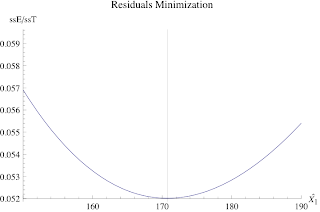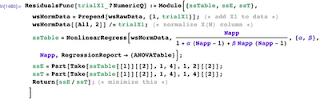## Tuesday, July 29, 2008

### How to Recover the Missing X(1) for the USL Scalability Model

When it comes to assessing application scalability, controlled measurements of the type that can be obtained with tools like Grinder or LoadRunner, are very useful because they provide a direct measurement of the throughput, X(N), as a function of the vuser/generator load, N. These data can be input easily into my universal scalability model (USL). To apply USL, however, you need to normalize all the X(N) data to the X(1) value. It often happens that the X(1) value may not have been measured by your QA group or it simply may not be measurable easily. What do you do in that case? You estimate it (carefully).In Section 5.7.3 of my GCaP book, I explain how to use Excel to estimate X(1) from a fit to Amdahl's law. In that case, you only have to fit a single modeling parameter, whereas the USL requires fitting two modeling parameters. In the upcoming GDAT class, we will show you how to do this more accurately using nonlinear regression analysis based on iteratively minimizing the residuals (shown above) in both R and Mathematica. You really need to use these more sophisticated techniques to avoid numerical accuracy problems that might be lurking in Excel.

In setting up this discussion for the GDAT class, I ran into a frustrating problem with Mathematica. Here's the code from Jim's R script ...R has a function called optimize() which successively calls a user-defined function, function(x), with new trial values of X(1) until it determines the minimum value of sse/sst (the ratio of the estimated sum-of-squares to the total) viz., the bottom of the curve shown above. The latter values are calculated using the nls (nonlinear least-squares) library function in R with the USL model as its argument (the thing with the 'sigma' and 'lambda' in it; using my old notation). David Lilja will explain the role of SSE and SST in the first part of the class.

You can do exactly the same thing in Mathematica and here is my equivalent user-defined function:Slight problem. The call-back didn't work! The argument trialX1 was not being evaluated, so the NonlinearRegress function (the Mathematica equivalent of "nls") barfed. After a lot of debugging, I finally discovered the problem. Long story short: unlike R, Mathematica can also do symbolic computations. In fact, that's its primary use (don't you wish you had it to evaluate those nasty integrals in your Calculus class?). Because of this "bias", it first tries to evaluate the argument trialX1 as a symbol, rather than a number. This behavior is correct but the justification is technical, so I won't go into that here. To disuade Mathematica from doing that, I needed to tell it explicitly to treat the argument as a numeric type and that's what the incantation trialX1_?NumericQ does. Simple, when you know!

Once again, this proves what I've said on many occasions: All modeling is programming and all programming is debugging.